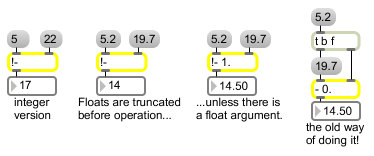# !-

Subtraction object (inlets reversed)

## Description

The !- object functions just like the - object, but the inlets' functions are reversed.

## Arguments

Name Type Opt Description
initial-subtraction-value int or float opt Sets the initial value, to be subtracted from a number received in the left inlet. A float argument causes the numbers to be subtracted as floats.

## Messages

 bang In left inlet: Performs the subtraction with the numbers currently stored. If there is no argument, !- initially holds 0. int input [int] In left inlet: The number is stored, and will be subtracted from a number received in the right inlet. (inlet1) input [int] In right inlet: The number in the left inlet is subtracted from the number, and the result is sent out the outlet. float input [float] Converted to int, unless !- has a float argument. (inlet1) input [float] Converted to int, unless !- has a float argument.

## Output

float: Only if there is an argument with a decimal point.
int: The difference between the two numbers received in the inlets.

## Examples- with the inputs swapped